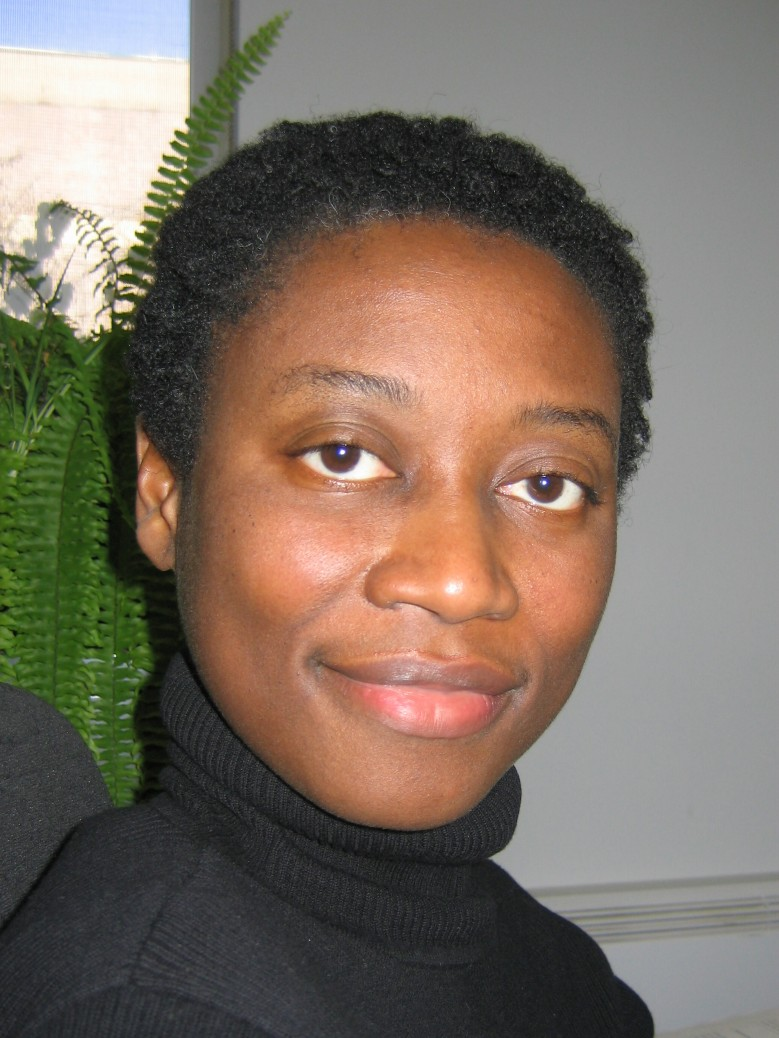# Lucy Campbell's web pages## Research

My research interests are in geophysical fluid dynamics, nonlinear waves, numerical methods for partial differential equations, and asymptotic and perturbation methods.

I am an Associate Editor for the Canadian Journal of Mathematics and Canadian Mathematical Bulletin.

I was an Associate Editor for the journal Mathematics of Climate and Weather Forecasting.

## Teaching

Fall 2021:

Precalculus: Functions and Graphs (MATH 0005A)
Ordinary Differential Equations (MATH 5405)

Winter 2022:

Ordinary Differential Equations (MATH 3008)
Numerical Analyis for Differential Equations (MATH 4816/5806)